# [Problem Set 3 Spike trains] Problem 3: Integrate-and-Fire neuron

Link of the iPython notebook for the code

# PROBLEM 3: Integrate-and-Fire neuron

We will now:

• first study the integrate-and-fire model with a constant input current, to acount for the creation of action potential (spikes) in real neurons

• secondly make the input current vary over time, to try to account for the spike trains that were experimentally measured in the primary somatosensory cortex of a monkey experiencing a vibratory stimulus on the finger (cf. the previous problem)

## 1. Integrate-and-Fire neuron with constant input current

### Voltage accross a passive membrane

The voltage across a passive neuron’s membrane when a current $I$ is injected is given by:

C \frac{ {\rm d}V}{ {\rm d}t} = g_L (E_L - V(t))+I

where

• $C = 1 \text{ nF}$ is the membrane capacitance
• $g_L = 0.1 \text{ μS}$ is the leak conductance of the membrane
• and $E_L = −70 \text{ mV}$ its reversal potential

On top of that, we will set

• $I$ to be equal to $1 \text{ nA}$
• $V(0)$ to be equal to $E_L$

The Euler method yields:

\begin{align*} & \; C \frac{ ΔV}{ Δt} = g_L (E_L - V(t))+I\\ ⟺ & \; \frac{ ΔV}{ Δt} = \frac 1 C (g_L (E_L - V(t))+I)\\ ⟺ & \; V(t+Δt) - V(t) = \frac {Δt} C (g_L (E_L - V(t))+I)\\ ⟺ & \; V(t+Δt) = \Big(1- \frac {Δt \cdot g_L} C\Big) V(t) + \frac {Δt \, (g_L \, E_L + I)} C \end{align*}

which enables us, by setting $∆t = 1 \text{ ms}$, to plot the voltage until time $t = 100 \text{ ms}$ for instance: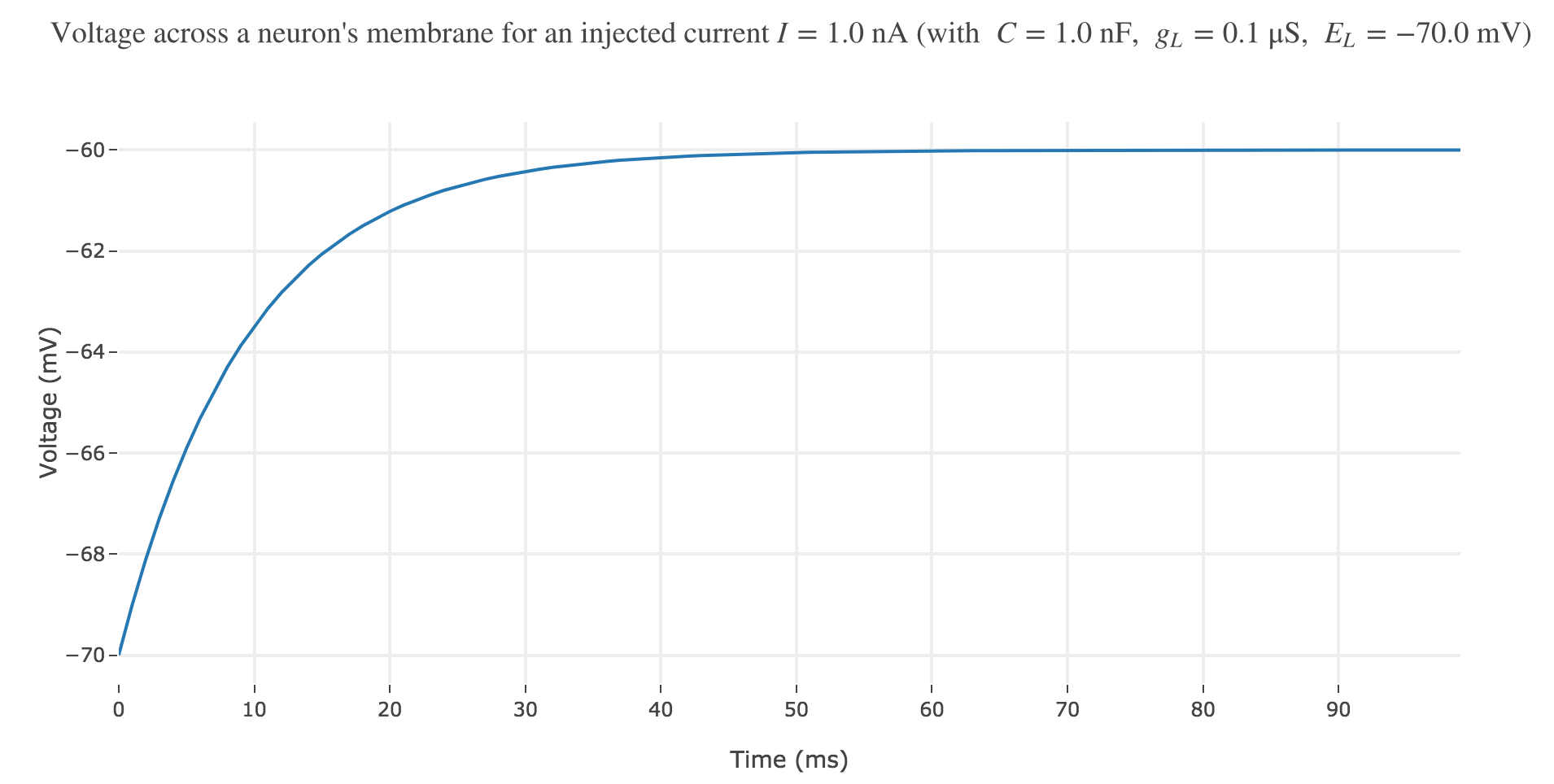Figure a. - Voltage accross a neuron's passive membrane for an injected current $I=1 \text{ nA}$

Now, for various current inputs $I$: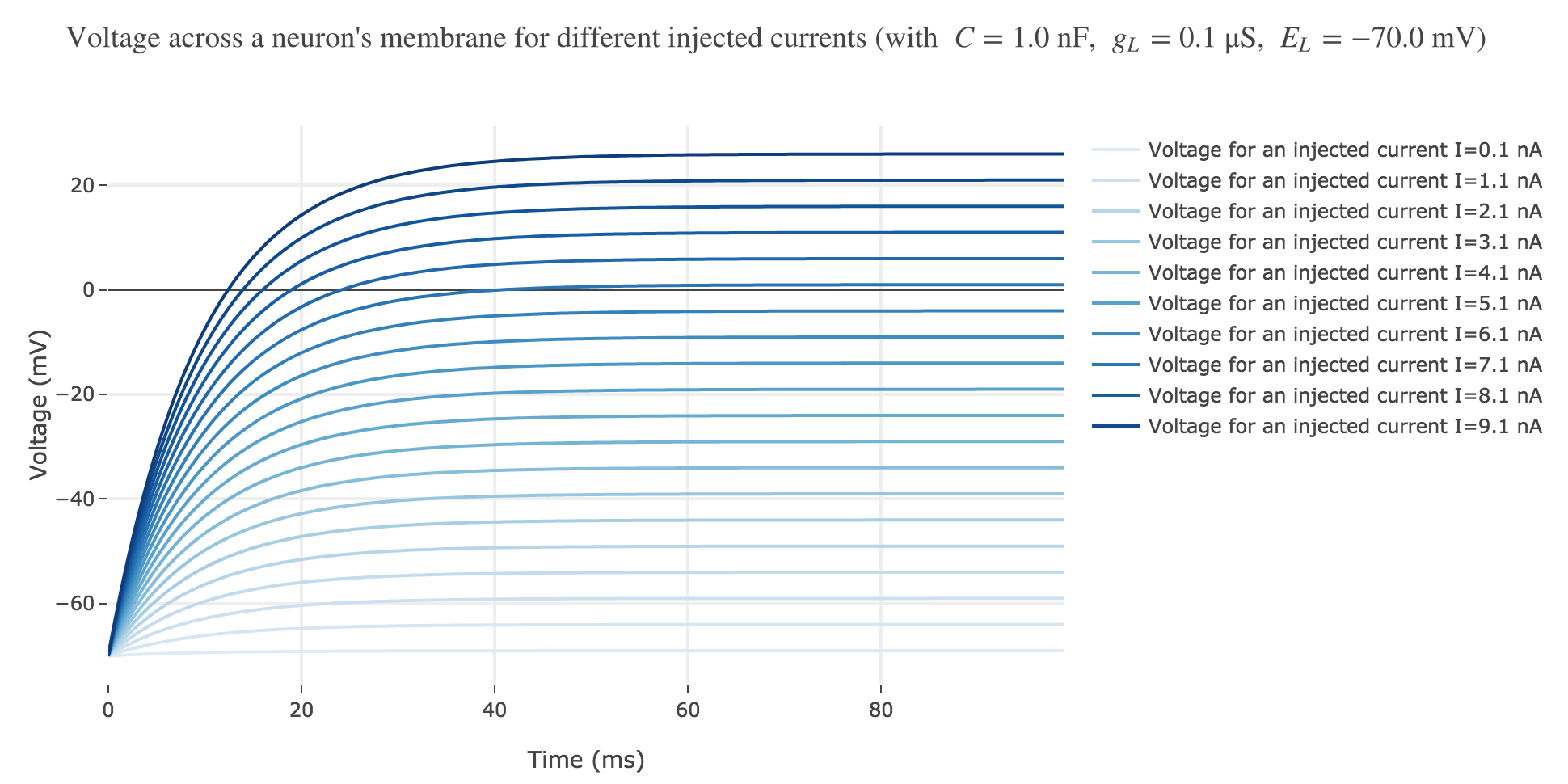Figure b.1. - Voltage accross a neuron's passive membrane for positive different injected currents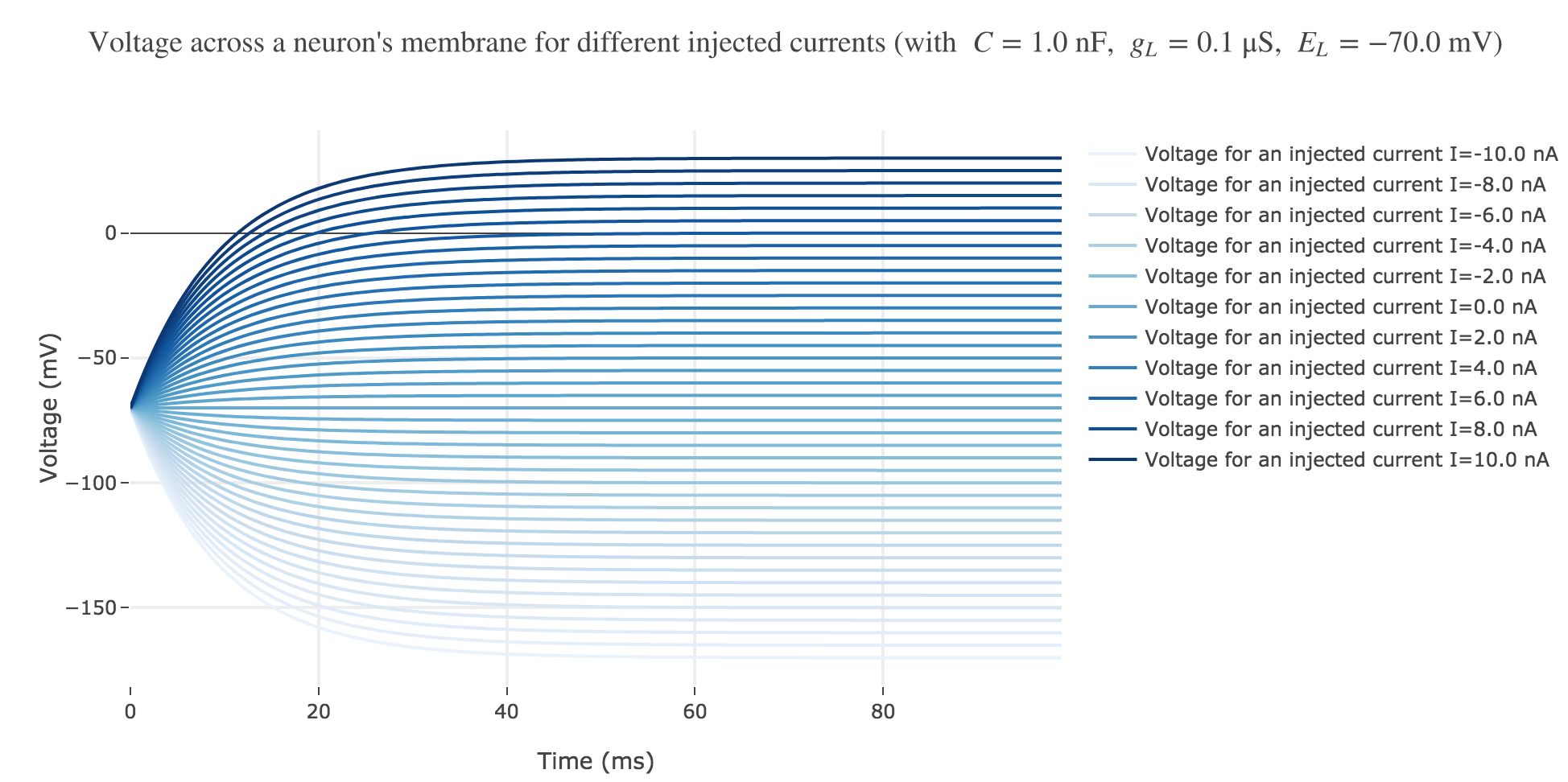Figure b.2. - Voltage accross a neuron's passive membrane for different positive and negative injected currents

For positive currents: It appears that the voltage accross the passive membrane is a non-decreasing monotonous function of the time (reaching a plateau after $40$ ms in our case) which increases when the input current does so.

For the negative currents: the curves are symmetric to the positive current ones with respect to the $V = E_L$ line.

To be more precise, let us solve for $V$ analytically:

\begin{align*} & \; \frac{ {\rm d}V}{ {\rm d}t} = \frac {g_L} C (E_L - V(t))+I/C\\ ⟺ & \; \frac{ {\rm d}V}{ {\rm d}t} + \frac {g_L} C V(t) = \frac {g_L \, E_L +I} C\\ ⟺ & \; \frac{ {\rm d}V}{ {\rm d}t} \, {\rm e}^{\frac {g_L \, t} C} + \frac {g_L} C V(t) \, {\rm e}^{\frac {g_L \, t} C} = \frac {g_L \, E_L +I} C \, {\rm e}^{\frac {g_L \, t} C}\\ ⟺ & \; \frac{ {\rm d}}{ {\rm d}t} \left[V(t) \, {\rm e}^{\frac {g_L \, t} C}\right] = \frac {g_L \, E_L +I} C \, {\rm e}^{\frac {g_L \, t} C}\\ ⟺ & \; V(t) \, {\rm e}^{\frac {g_L \, t} C} = \frac C {g_L} \frac {g_L \, E_L +I} C \, {\rm e}^{\frac {g_L \, t} C} + \text{const}\\ ⟺ & \; V(t) \, {\rm e}^{\frac {g_L \, t} C} = \left(E_L + \frac I {g_L}\right) \, {\rm e}^{\frac {g_L \, t} C} + \text{const}\\ ⟺ & \; V(t) \, = \left(E_L + \frac I {g_L}\right) + \text{const} × {\rm e}^{-\frac {g_L \, t} C}\\ \end{align*}

And with $V(0) = E_L$:

∀t ≥0, \quad V(t) \, = E_L + \frac I {g_L}\left(1-{\rm e}^{-\frac {g_L \, t} C}\right)

The symmetry with respect to the $V = E_L$ between negative and positive current curves is clearly seen in this analytical expression.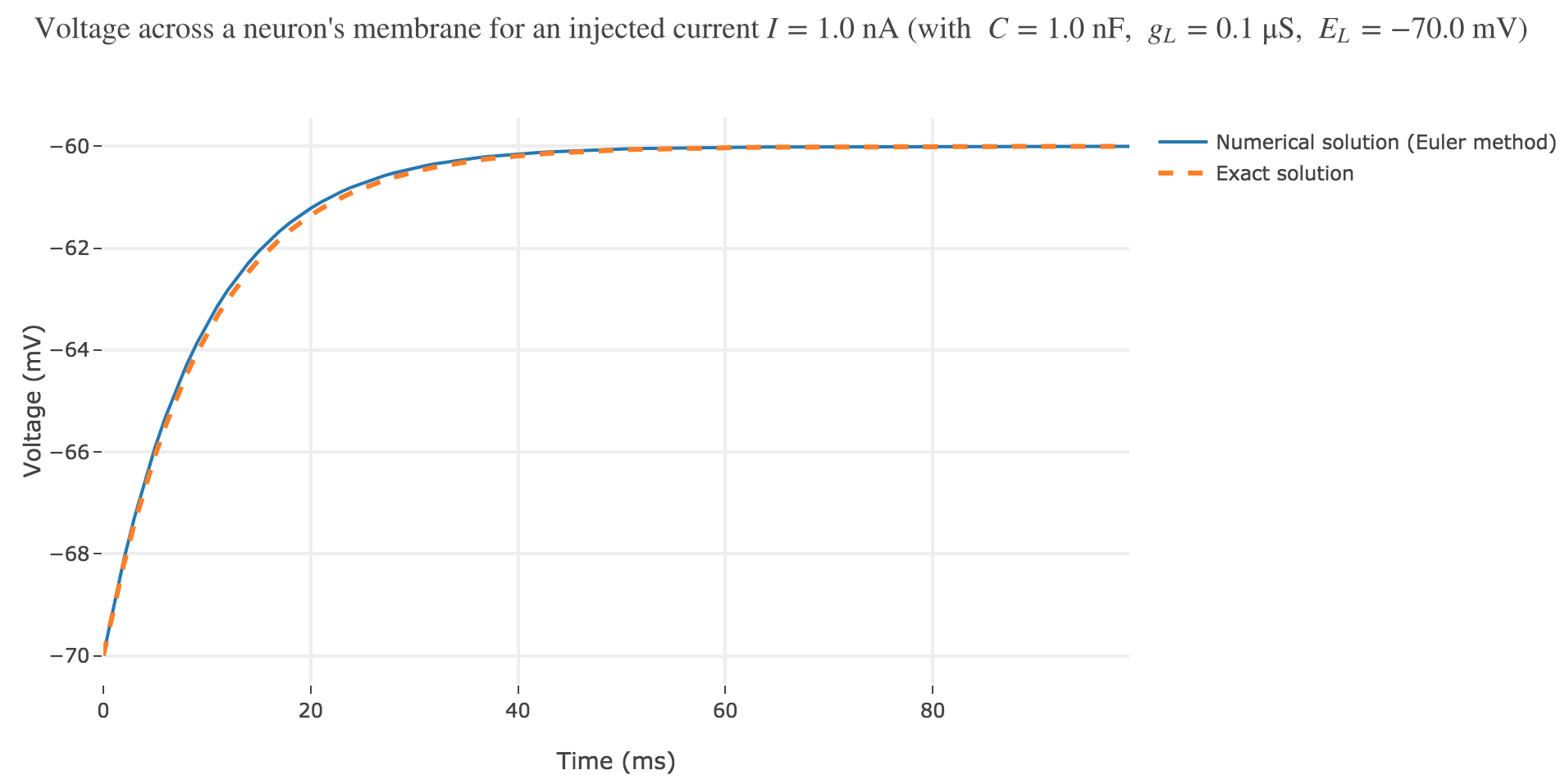Figure c. - Voltage accross a neuron's membrane: Comparison between the numerical solution and the exact one

As seen in Figure c. the numerical solution resulting from the Euler method and the exact one closely match.

So, on the whole:

• $V$ starts at $E_L$
• and then increases monotonously until $E_L + I/g_L$ (so the higher the input current, the higher the voltage, as examplified in Figure b)
• the characteristic time constant thereof is $C/g_L = 10 \text{ ms}$
• the derivative of $V$:

\frac{ {\rm d}V}{ {\rm d}t} ≝ \frac{I}{C} \, {\rm e}^{-\frac {g_L \, t} C}

is positively proportional to $I$: the higher the input current, the steeper the increase of $V$.

### Action-potential-generating mechanism

To account for spike generation, let us assume that the neuron fires each time $V$ gets greater than a threshold $V_{th}$, after which the membrane voltage is reset to its initial value $E_L$. Let us set $V_{th} ≝ −63 \text{ mV}$: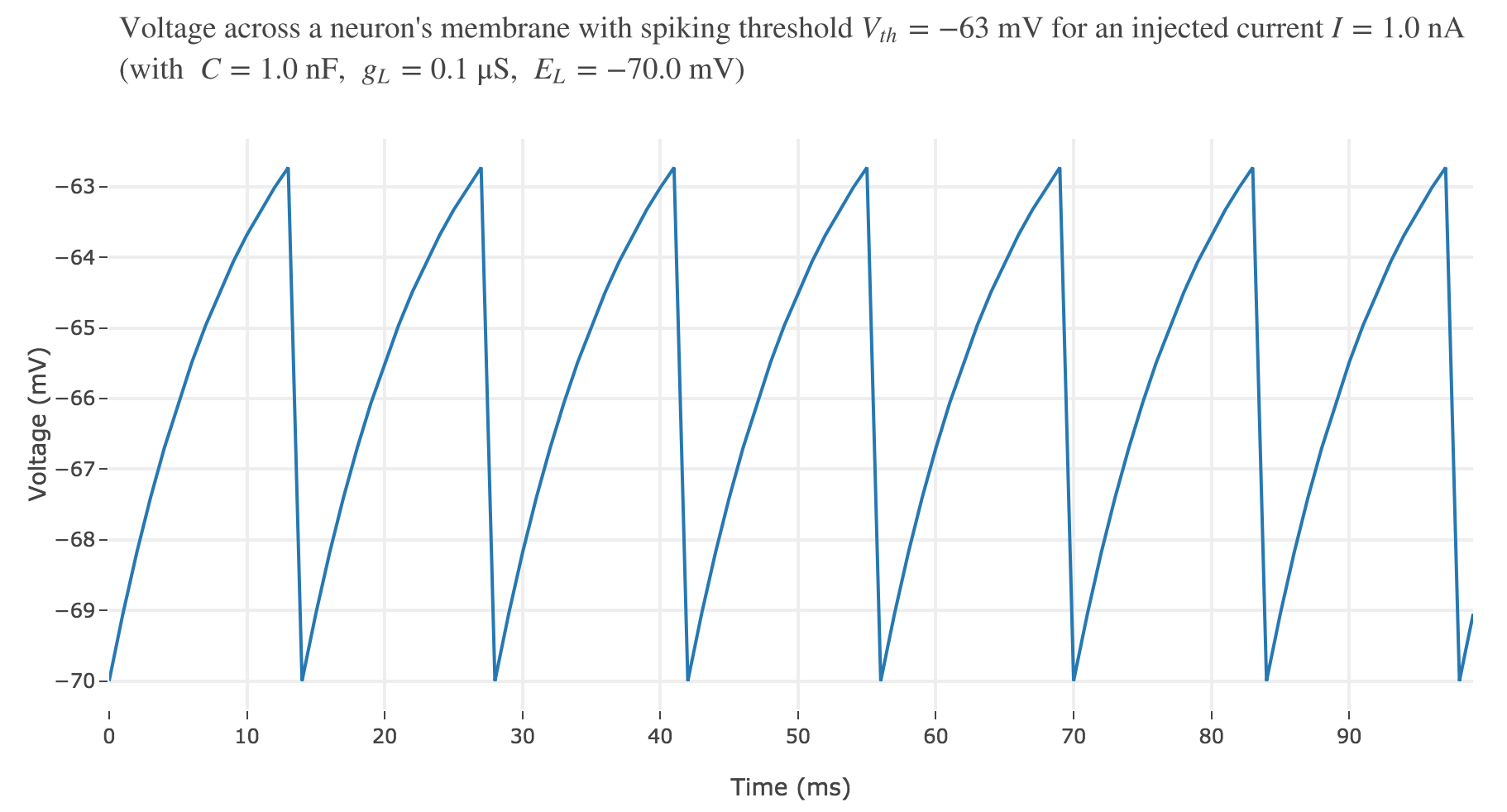Figure d.1. - Voltage accross a neuron's membrane with spiking threshold $V_{th} ≝ −63 \text{ mV}$ and an injected current of $1$ nA.

For an input current of $1$ nA, we get $7$ spikes within the first $100$ ms. Let us investigate how the number of spikes varies with the input current: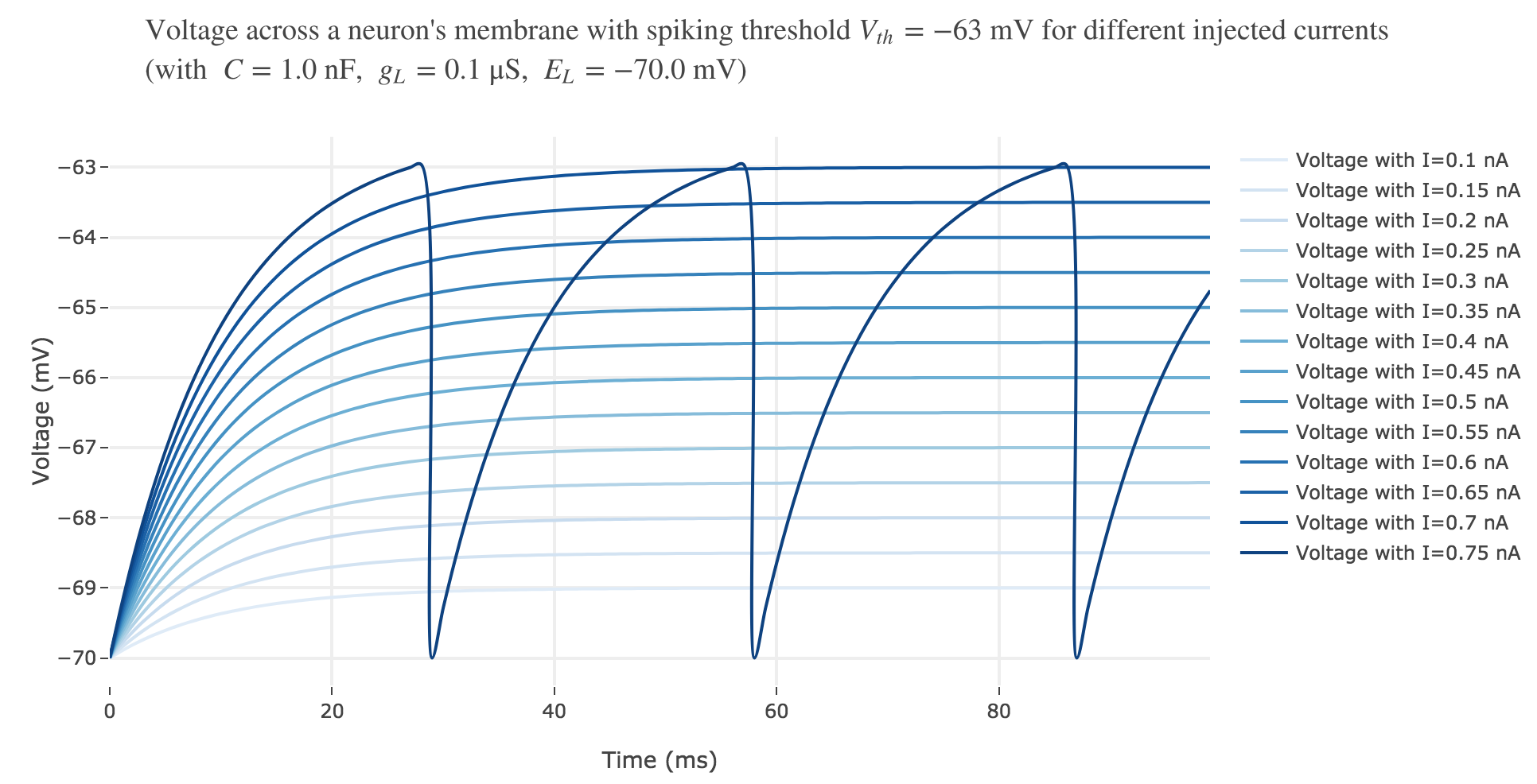Figure d.2. - Voltage accross a neuron's membrane with spiking threshold $V_{th} ≝ −63 \text{ mV}$ and different injected currents $≤ 0.75$ nA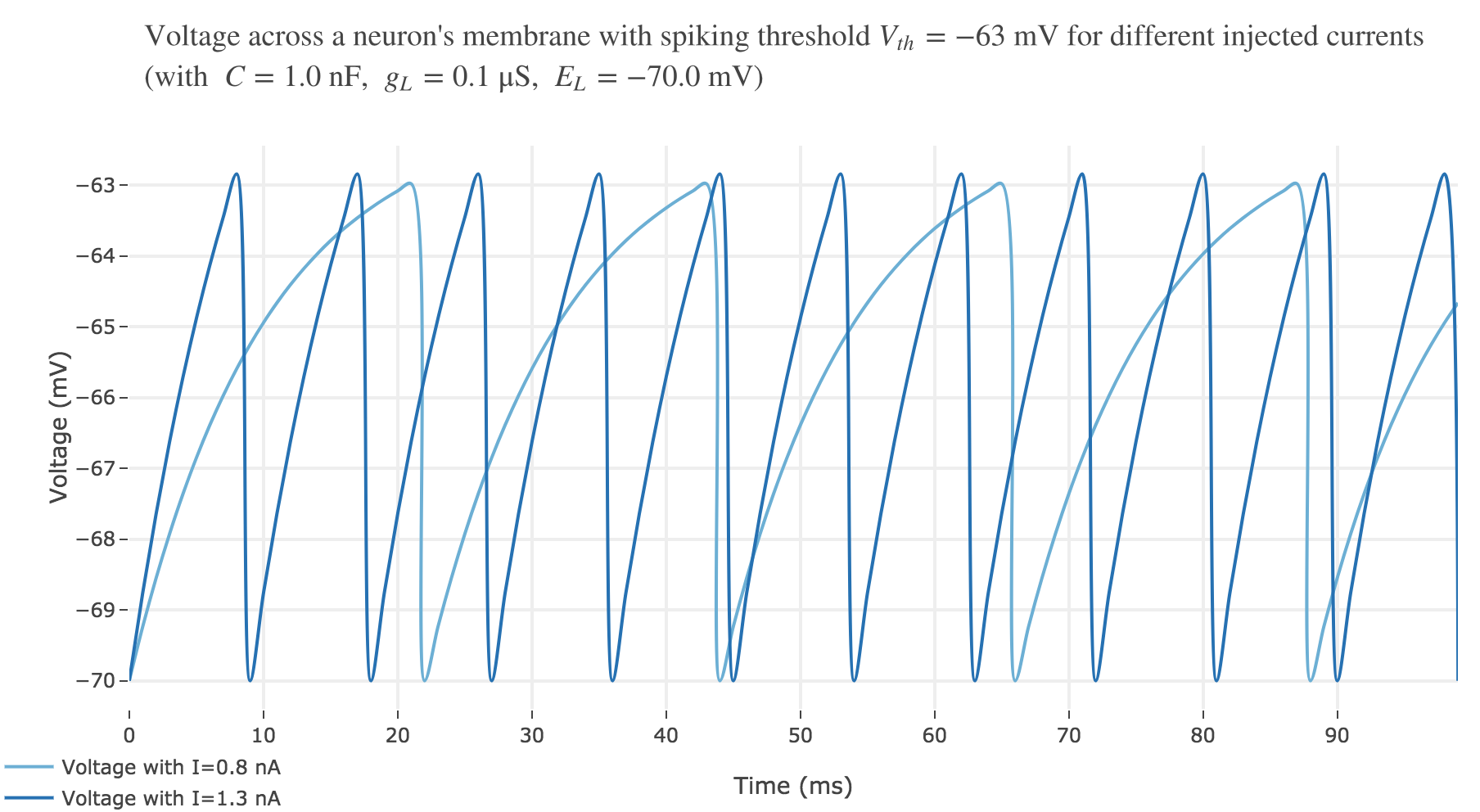Figure d.3. - Voltage accross a neuron's membrane with spiking threshold $V_{th} ≝ −63 \text{ mV}$ and injected currents $0.8$ and $1.3$ nA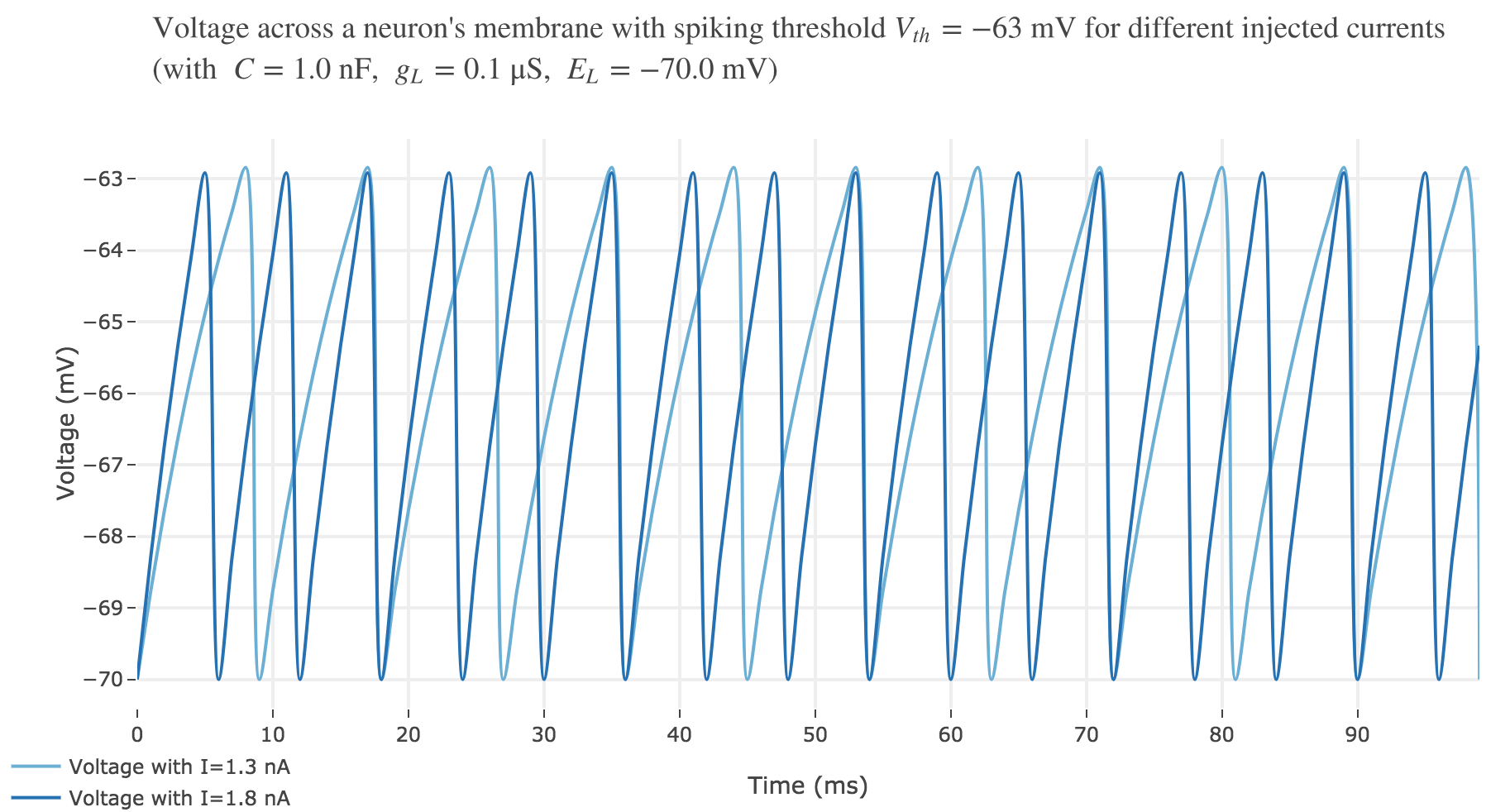Figure d.4. - Voltage accross a neuron's membrane with spiking threshold $V_{th} ≝ −63 \text{ mV}$ and injected currents $1.3$ and $1.8$ nA

For $I ≥ 0.75$ nA: The higher the input current, the steeper the increase of $V$ (as shown before) and hence the the faster $V$ reaches the threshold: so, as expected, we observe that the higher the input current, the higher the number of spikes within $100$ ms.

But for $I ≤ 0.7$ nA, no spike is fired. Indeed, the precise input current value at which the neuron start firing can be computed analytically:

\begin{align*} & \; ∃t; \; V(t) \, \overset{\text{eventually}}{>} V_{th}\\ ⟺ & \; \lim\limits_{t \to +∞} V(t) > V_{th} &&\text{ (as } V \text{ is non-decreasing)}\\ ⟺ & \; E_L + \frac I {g_L} > V_{th}\\ ⟺ & \; I > g_L (V_{th} - E_L) \end{align*}

As it happens, with with $E_L ≝ -70 \text{ mV}$ and $V_{th} = -63 \text{ mV}$:

∃ t; \quad V(t) \, \overset{\text{eventually}}{>} V_{th} ⟺ I > 0.7 \text{ nA}

Let us plot a rastergram of the spike trains for various injected currents, so as to to see more easily how the number of spikes is impacted: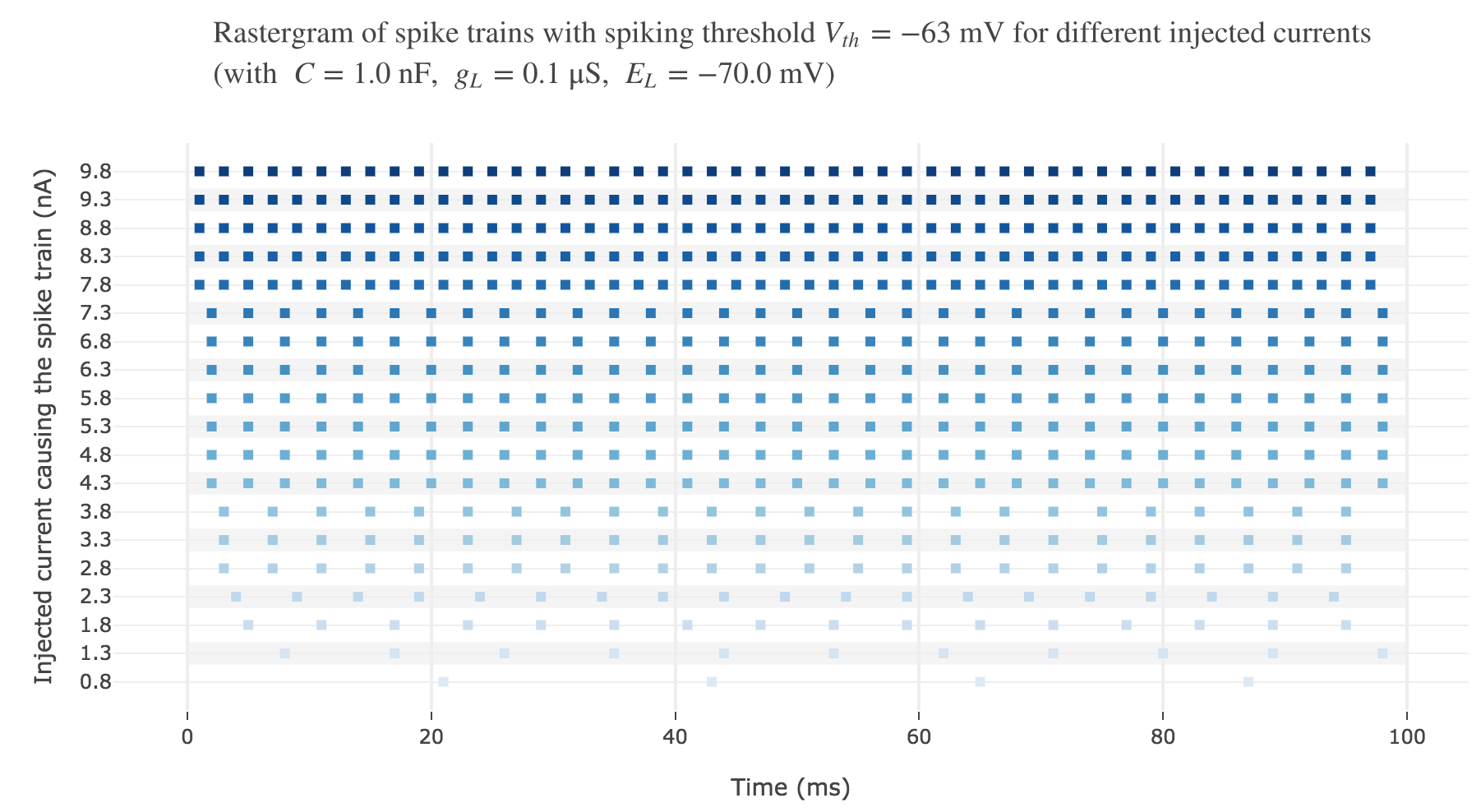Figure d.5. - Rastergram of spike trains with spiking threshold $V_{th} ≝ −63 \text{ mV}$ for various injected currents

For $I ≥ 7.8$ nA, the number of spikes reaches its maximum value of $49$ spikes within $100$ ms.

To make it even more clear, let us plot the tuning curve of this neuron (i.e. the number of spikes within $100 \text{ ms}$ depending on the input current $I$):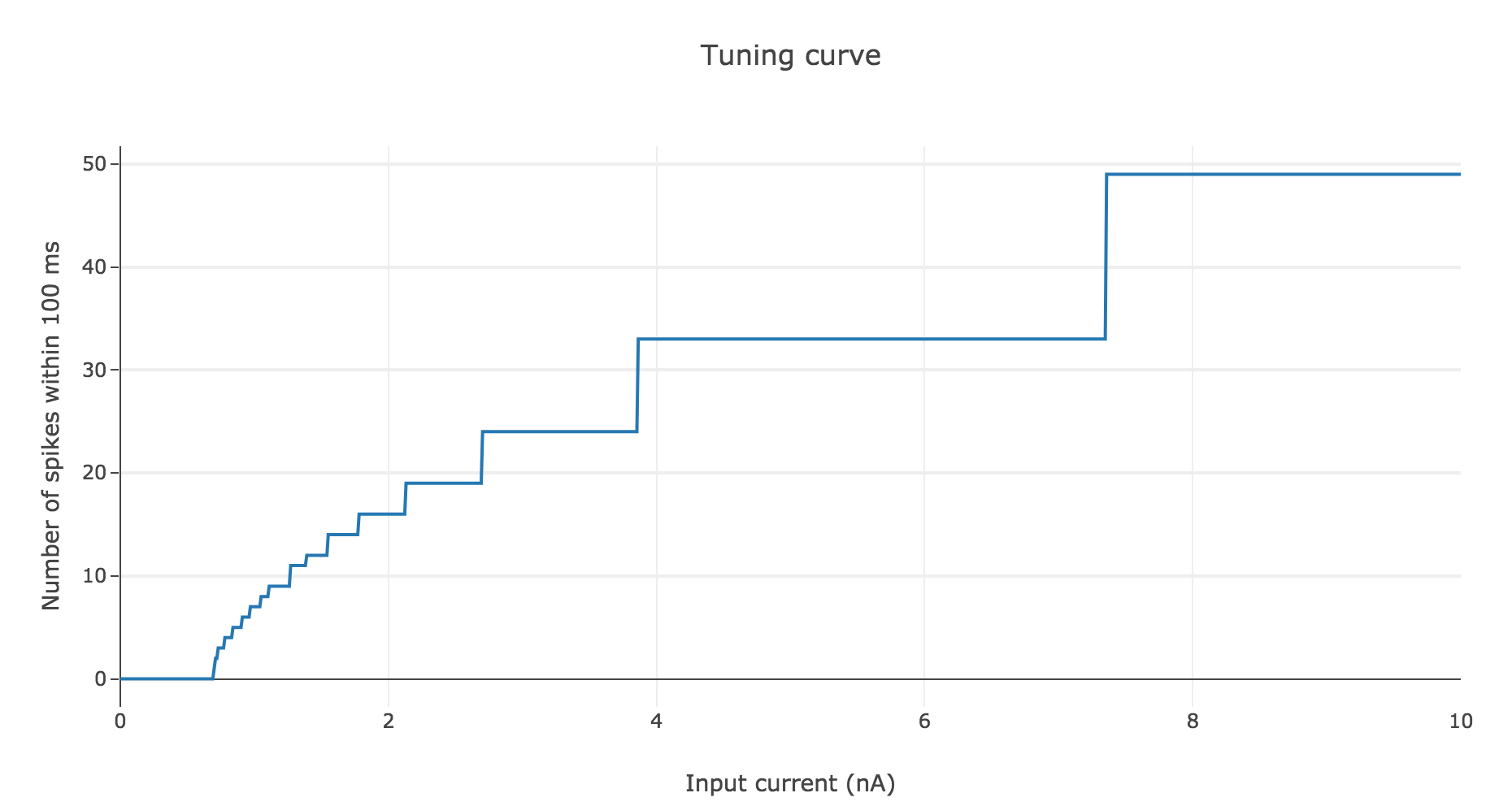Figure d.6. - Number of spikes within $100 \text{ ms}$ as a function of the input current $I$

As shown before, the current threshold at which the neuron starts firing is

I_{th} ≝ g_L (V_{th} - E_L)

This makes sense intuitively:

• the higher the difference $V_{th} - E_L$, the more the voltage $V$ has to increase to reach $V_{th}$ within $100 \text{ ms}$, and we saw previously that it requires a higher input current $I$

• the higher the leak conductance $g_L$ of the membrane, the lower the voltage $V$ by Ohm’s law (as $V$ is inversely proportional to the conductance), and the higher the input current is required to be, to compensate

### Refractory period

Whenever the neuron depolarize until the voltage hits the threshold: it fires, then the voltage is reinitialized at $E_L$, but it can be shown experimentally that the voltage doesn’t increase right away after having been reset: for a fixed time span (the refractory period), the voltage remains constant, then at the end of the refractory period it depolarizes again.

We can take into account this refractory period in our model: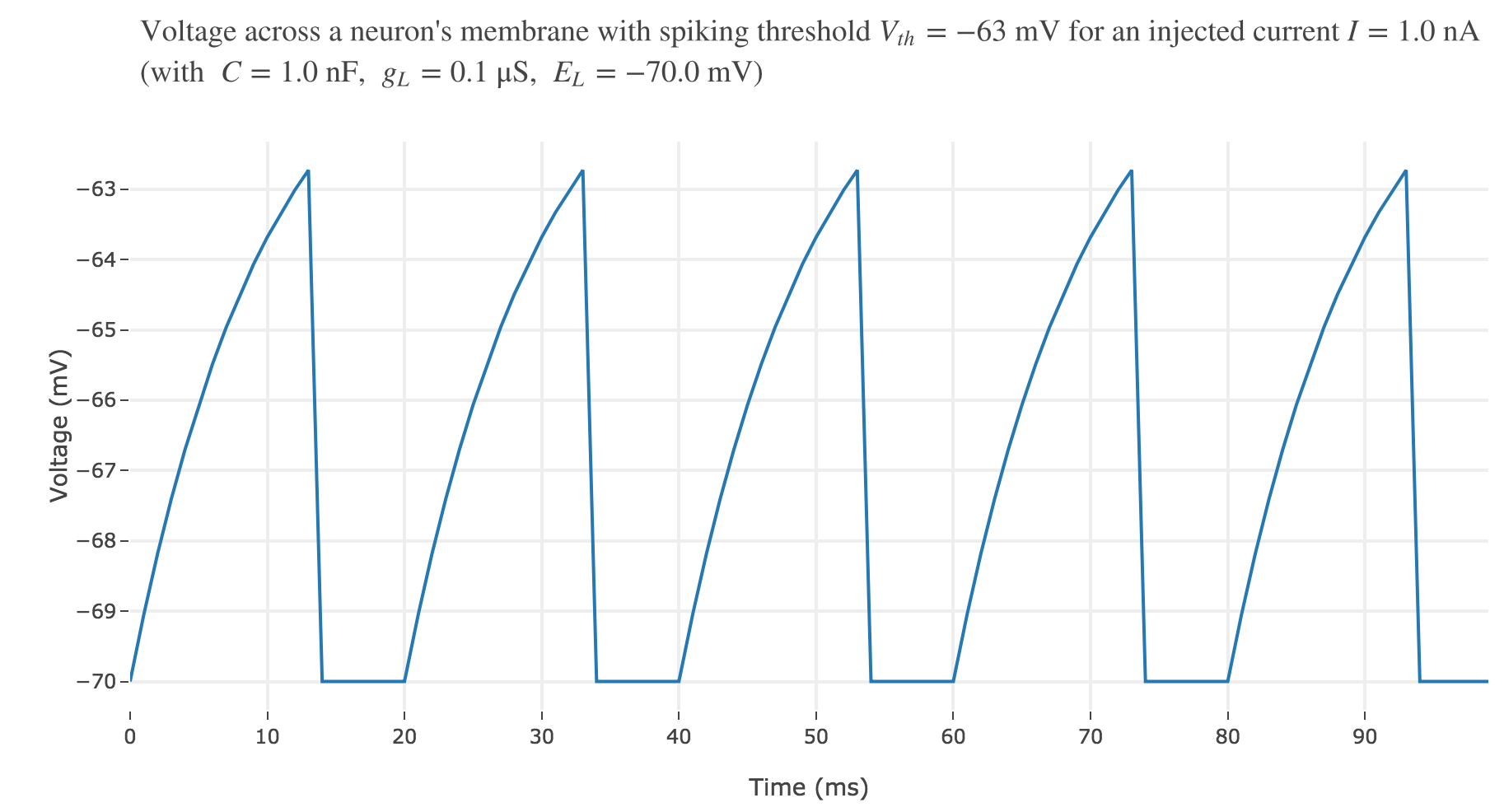Figure f.1. - Voltage accross a neuron's membrane with spiking threshold $V_{th} ≝ −63 \text{ mV}$ and a $1$ nA input current, having a $5 \text{ msec}$ refractory period.

On goes from $7$ spikes (before) to $5$ ones within the first $100$ ms, with a $5$ ms refractory period.

As expected: the higher the refractory period, the less spike the neuron fires within the same time span.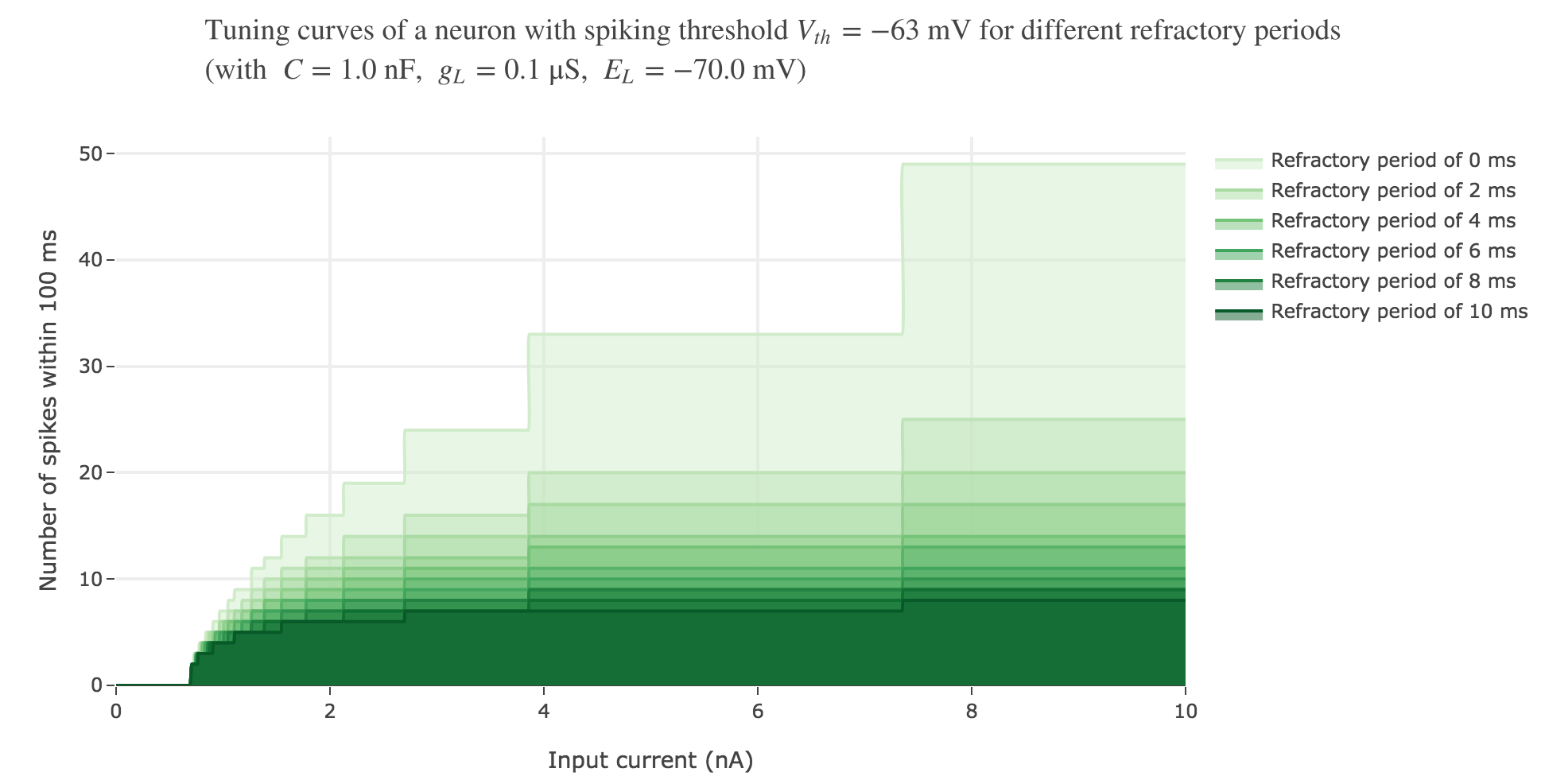Figure f.2. - Number of spikes within $100 \text{ ms}$ as a function of the input current $I$ for different refractory periods.

### With a white noise term

A more realistic model would take into account part of the noise there is in reality. A more noisy voltage accross a neuron’s passive membrane could be given by:

C \frac{ {\rm d}V}{ {\rm d}t} = g_L (E_L - V(t))+ I + ση(t)

where $σ$ is the noise magnitude and $η \sim 𝒩(0, 1)$. The choice of a Gaussian distribution is accounted for by the central limit theorem, since the noise can be thought of as a sum of many independent indentically distributed processes.

The Euler method yields:

C (V(t+ Δt) - V(t)) = g_L (E_L - V(t)) Δt + I Δt + σ \sqrt{Δt} \, η(t)

Thus:

V(t+Δt) = \Big(1- \frac {Δt \cdot g_L} C\Big) V(t) + \frac {Δt \, (g_L \, E_L + I)} C + \frac {σ \sqrt{Δt} \, η(t)} C

The $\sqrt{Δt}$ stems from the fact that

\sqrt{Δt} \, η(t) \sim 𝒩(0, Δt)

so that the noise magnitude amounts to $Δt$ when adding a $Δt$ time step.

The white noise term makes it look more realistic: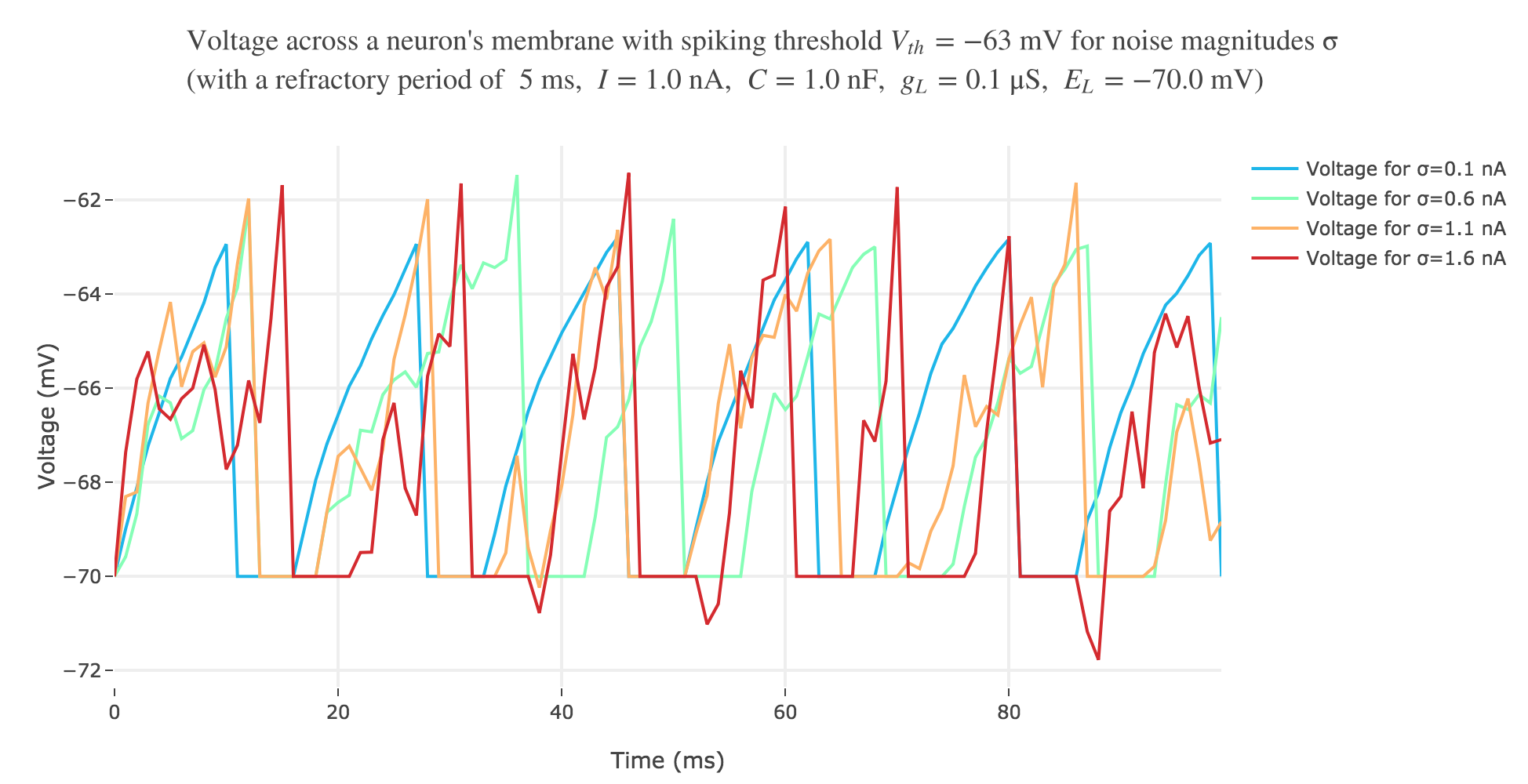Figure g.1. - Voltage accross a neuron's membrane with spiking threshold $−63 \text{ mV}$, a $1$ nA input current and a $5 \text{ msec}$ refractory period, for various noise magnitudes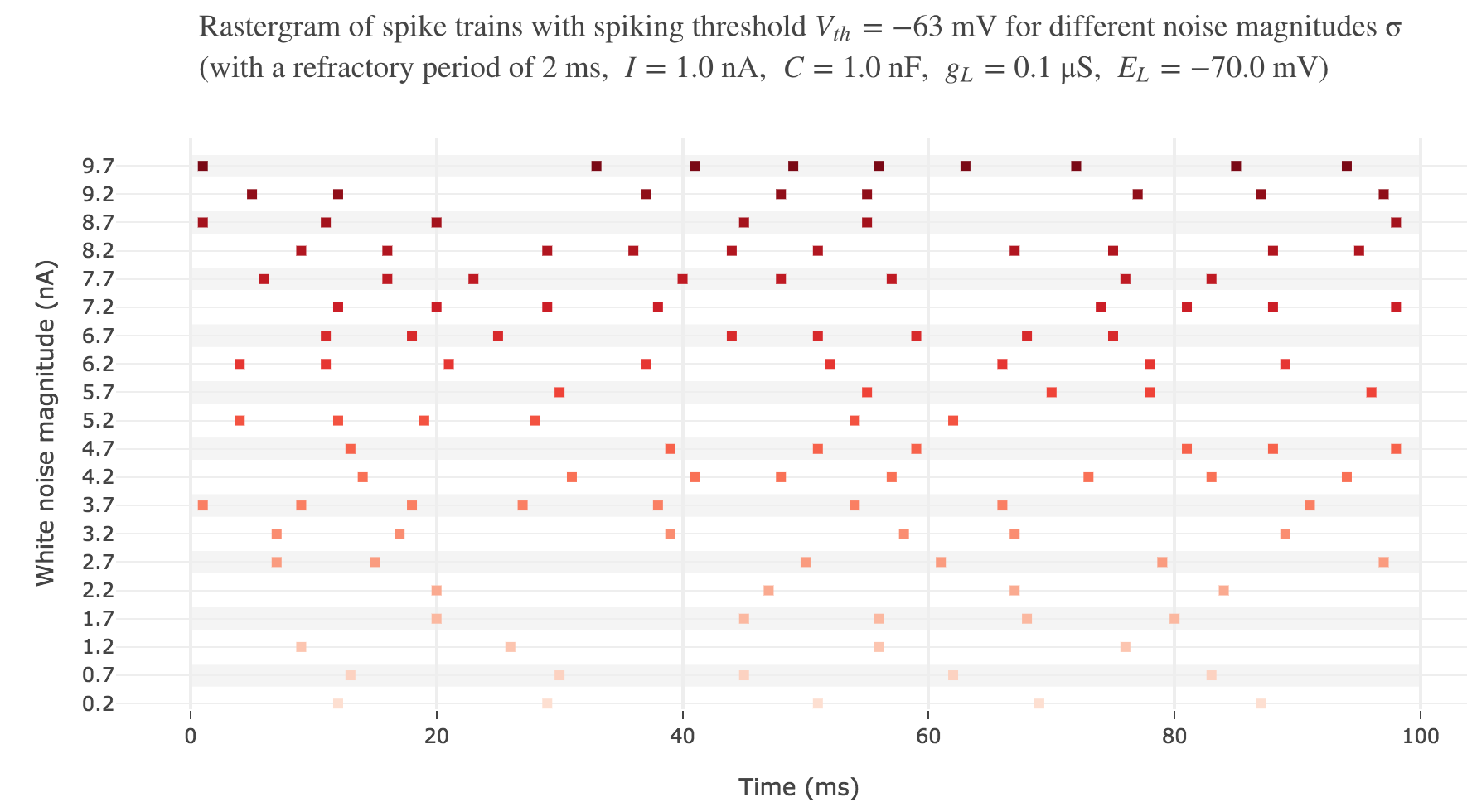Figure g.2. - Rastergram of spike trains with spiking threshold $−63 \text{ mV}$,a $1$ nA input current and a $5 \text{ msec}$ refractory period, for various noise magnitudes

## 2. Integrate-and-Fire neuron with time-varying input current to match experimental data

Finally, let us try to model the experminental spike trains generated by a given neuron in the primary somatosensory cortex of a monkey subject to a vibratory stimulus on the finger (as seen in the previous problem).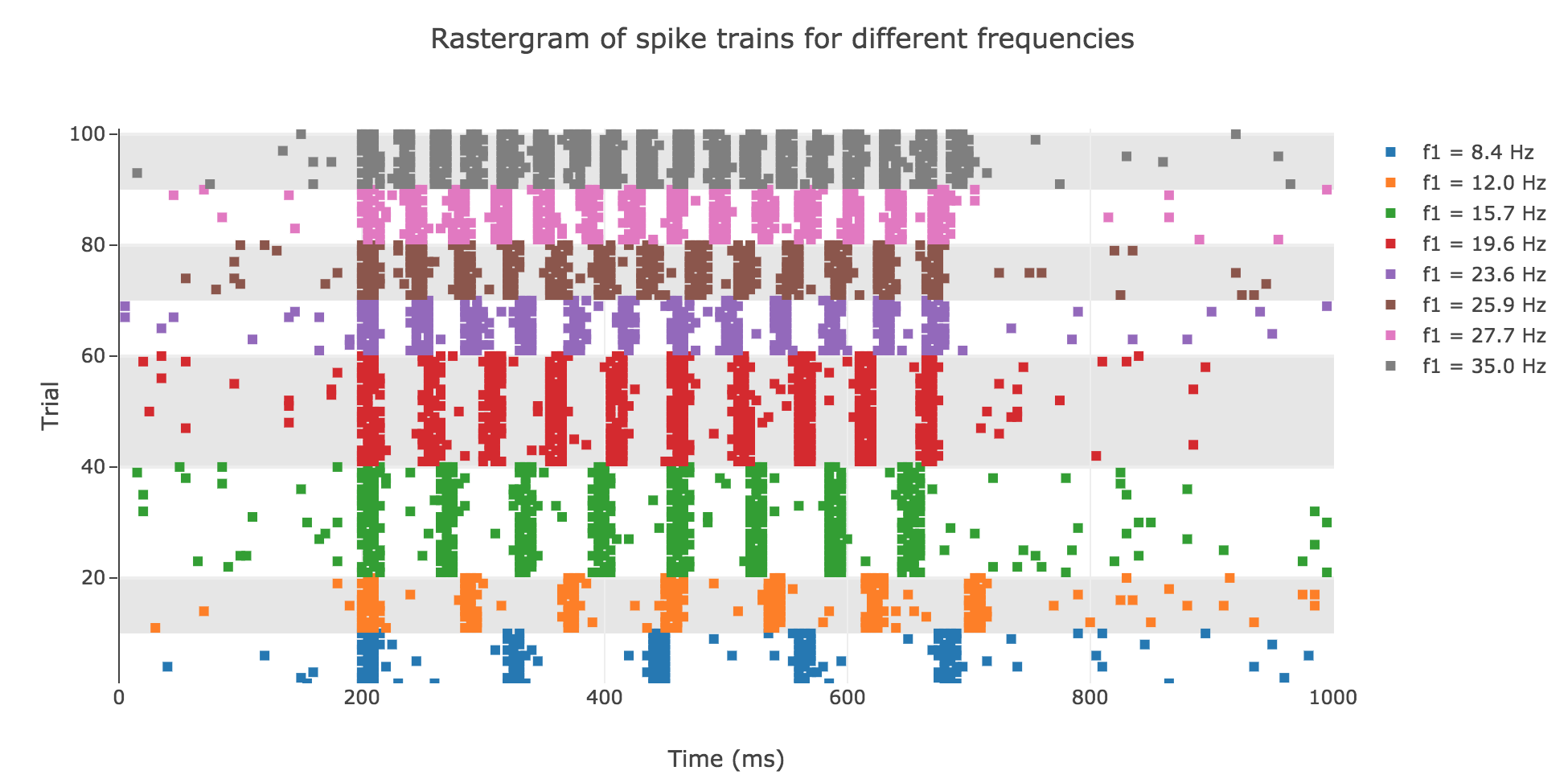Figure h.1. - Rastergram of the experimental spike trains for various vibration frequencies

To do so, a constant input current will not be enough, as shown in Figure g.2.: the rastergram is not likely to match what is observed experimentally. And above all, the vibration frequency $f_1$ has not even been taken into account so far, which cannot but lead us to fail to model the experimental spike trains, as we showed that there is a linear relationship between the average spike counts/firing rates and the vibration frequencies.

Looking at the experimental spike trains, we observed in the previous problem that the spikes seemed to occur periodically within the simulation time span (between $200$ and $700$ ms): the higher the vibration frequency, the smaller the interspike period.

This suggests a sinusoidal input current whose frequency is a multiple of the vibration frequency, modulated by an envelope that decays out of the simulation period.

### Gaussian envelope

With a Gaussian envelope, the time-varying input current

I(t) \propto \exp\left(-\frac{(t - μ)^2}{2 s^2}\right) \cos(f_1 (t-μ)/200)

where $μ = 450 \text{ ms}, \; s = 150 \text{ ms}$, could look like this: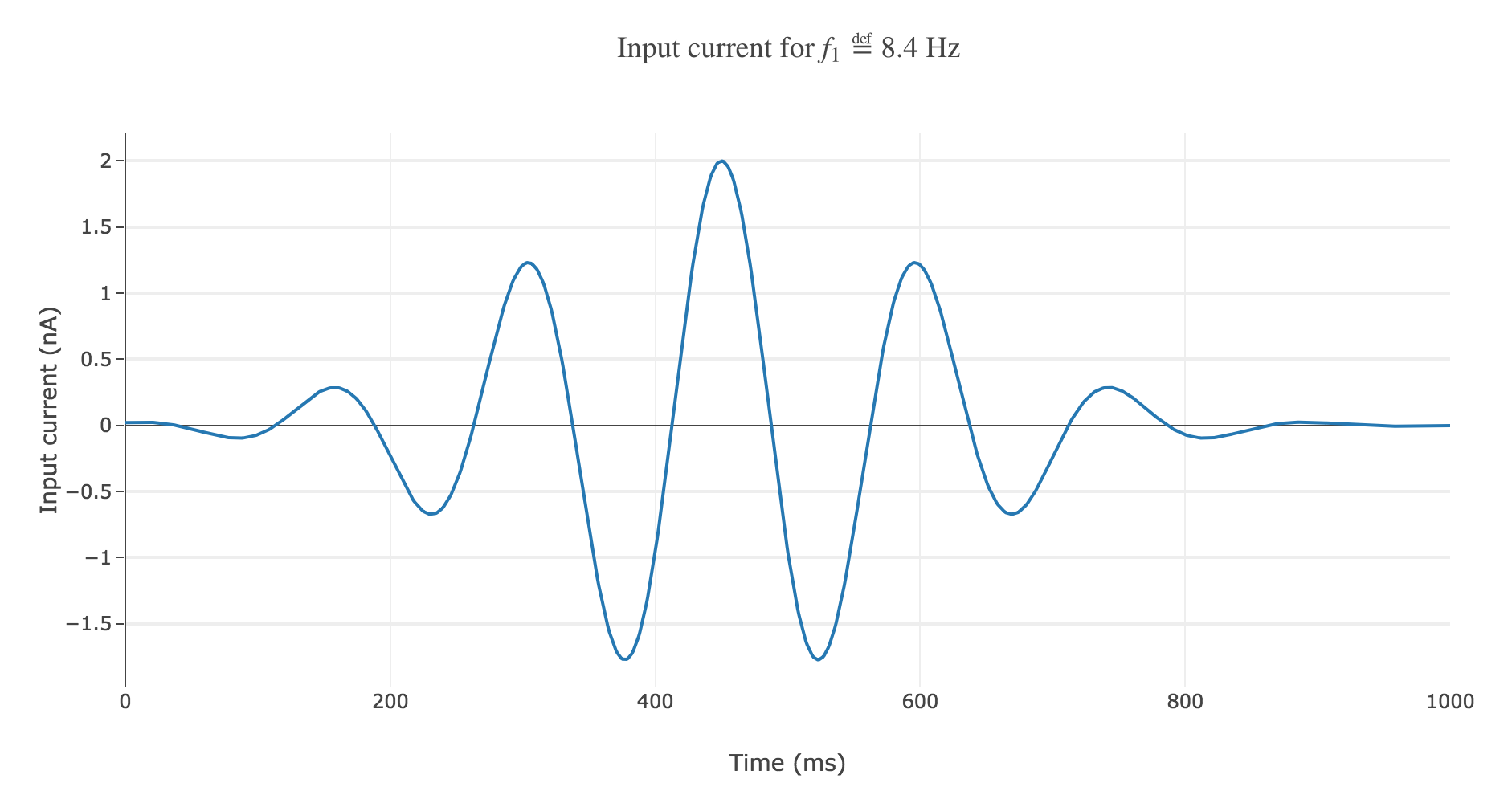Figure h.2. - Sinusoidal input current with a Gaussian envelope

It leads to the following rastergram: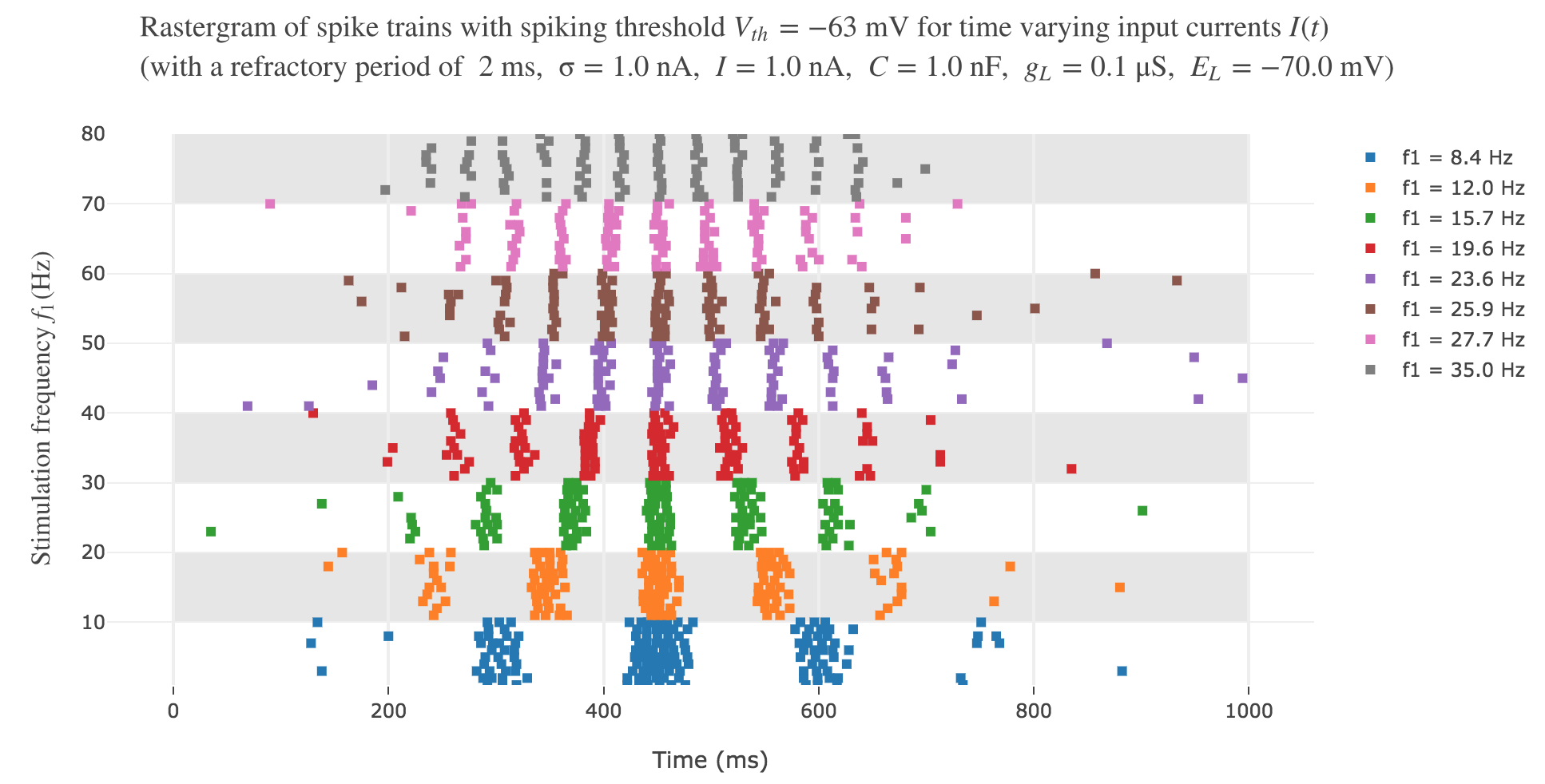Figure h.3. - Rastergram of artifical spike trains induced by a sinusoidal input current modulated by a Gaussian envelope, for various vibration frequencies

which is not satisfying, with regard to the experimental one.

### Heaviside envelope

Another solution would be to go for a Heaviside envelope: the current is purely sinusoidal within the simulation period, it is equal to zero everywhere else.

The resulting time-varying input current

I(t) \propto \begin{cases} \cos(f_1 (t-200)/200) \text{ if } t∈[200, 700] \text{ ms} \\ 0 \text{ else} \end{cases}

could look like this: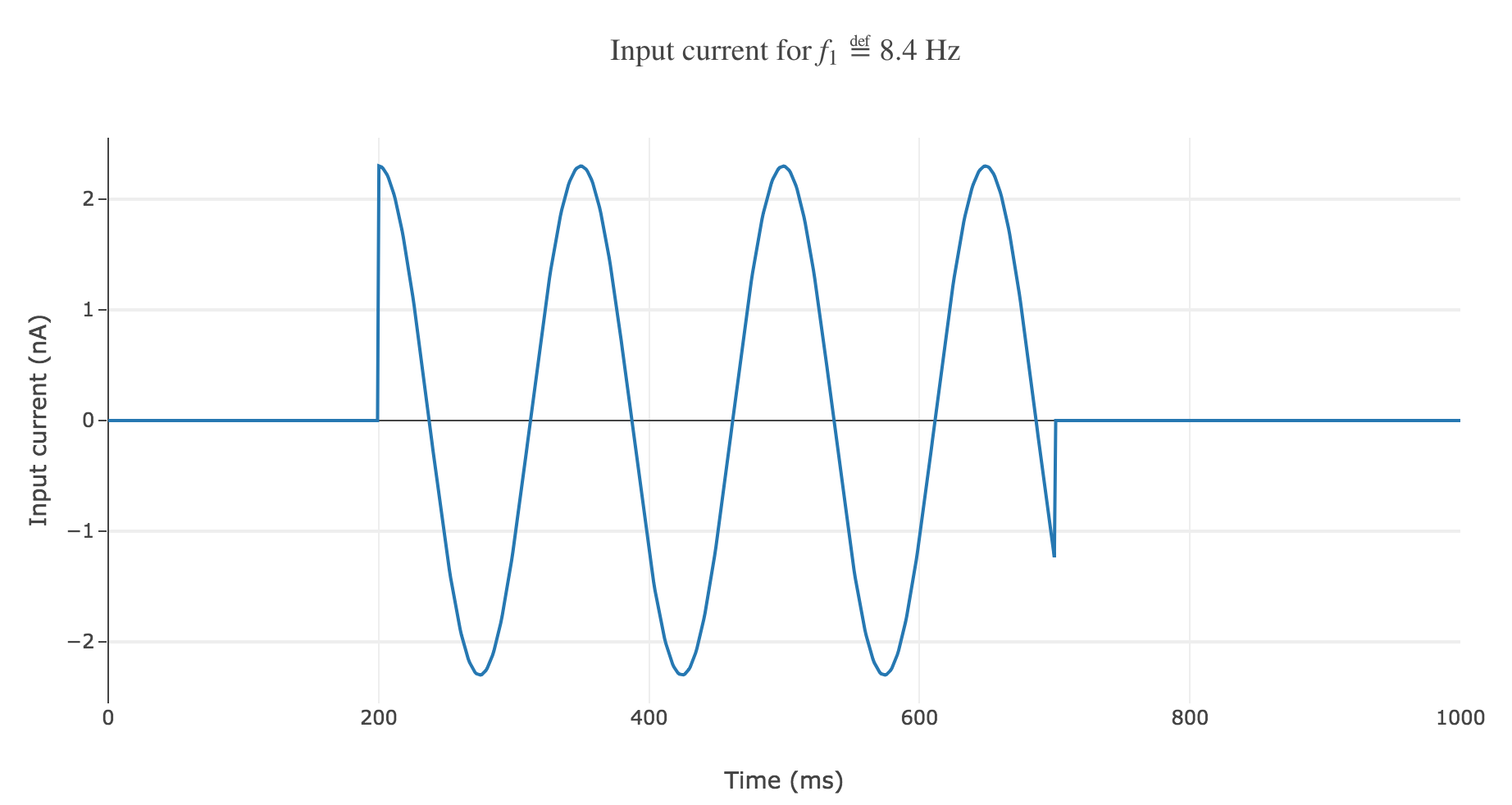Figure - Sinusoidal input current with a Heaviside envelope

The resulting rastergram: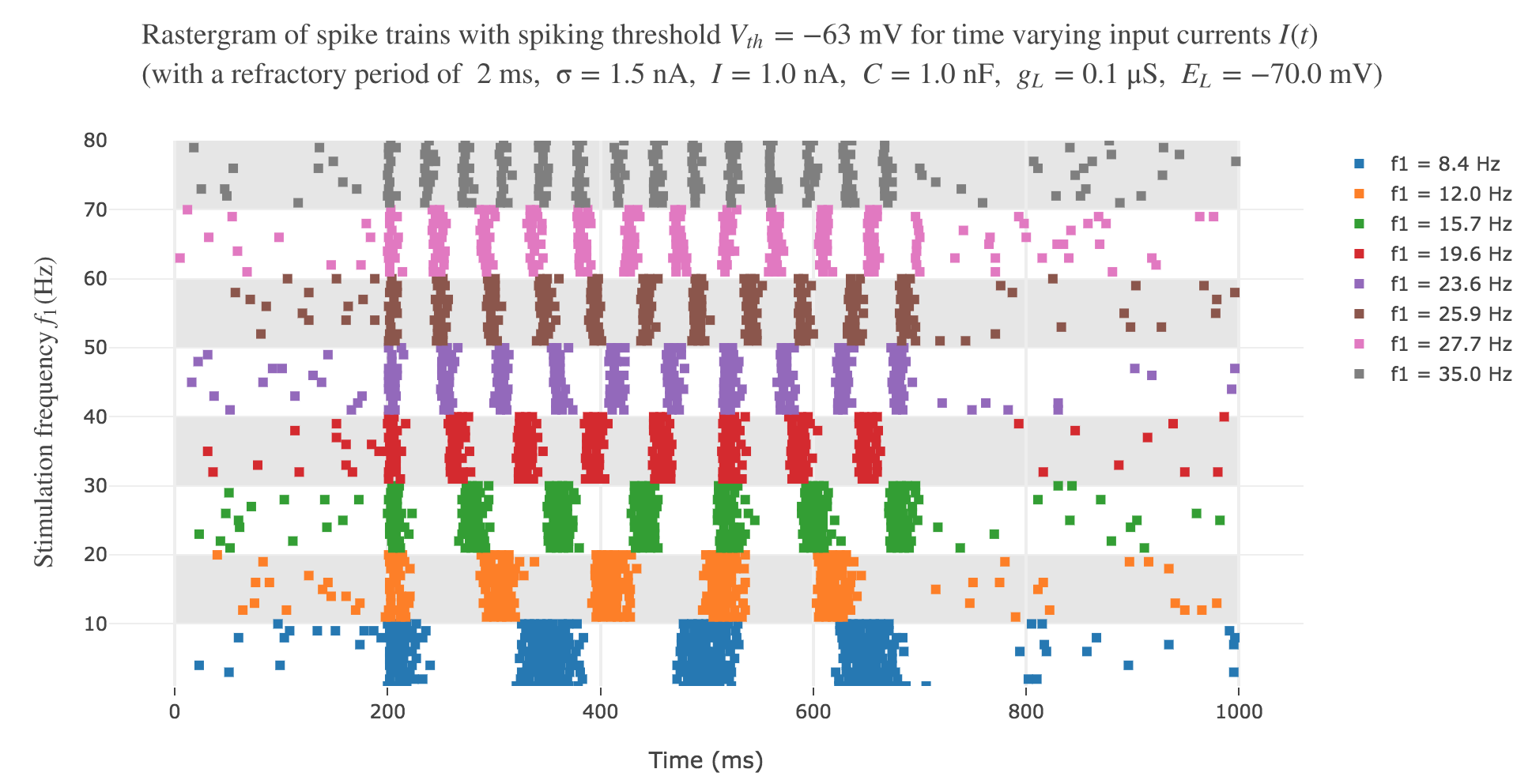Figure h.4. - Rastergram of artifical spike trains induced by a sinusoidal input current modulated by a Heaviside envelope, for various vibration frequencies

resembles way more to the experimental one!

However, the average spike count/firing rate as a function of the vibration frequency is not satisfying: it is somewhat anti-linear with respect to the vibration frequency!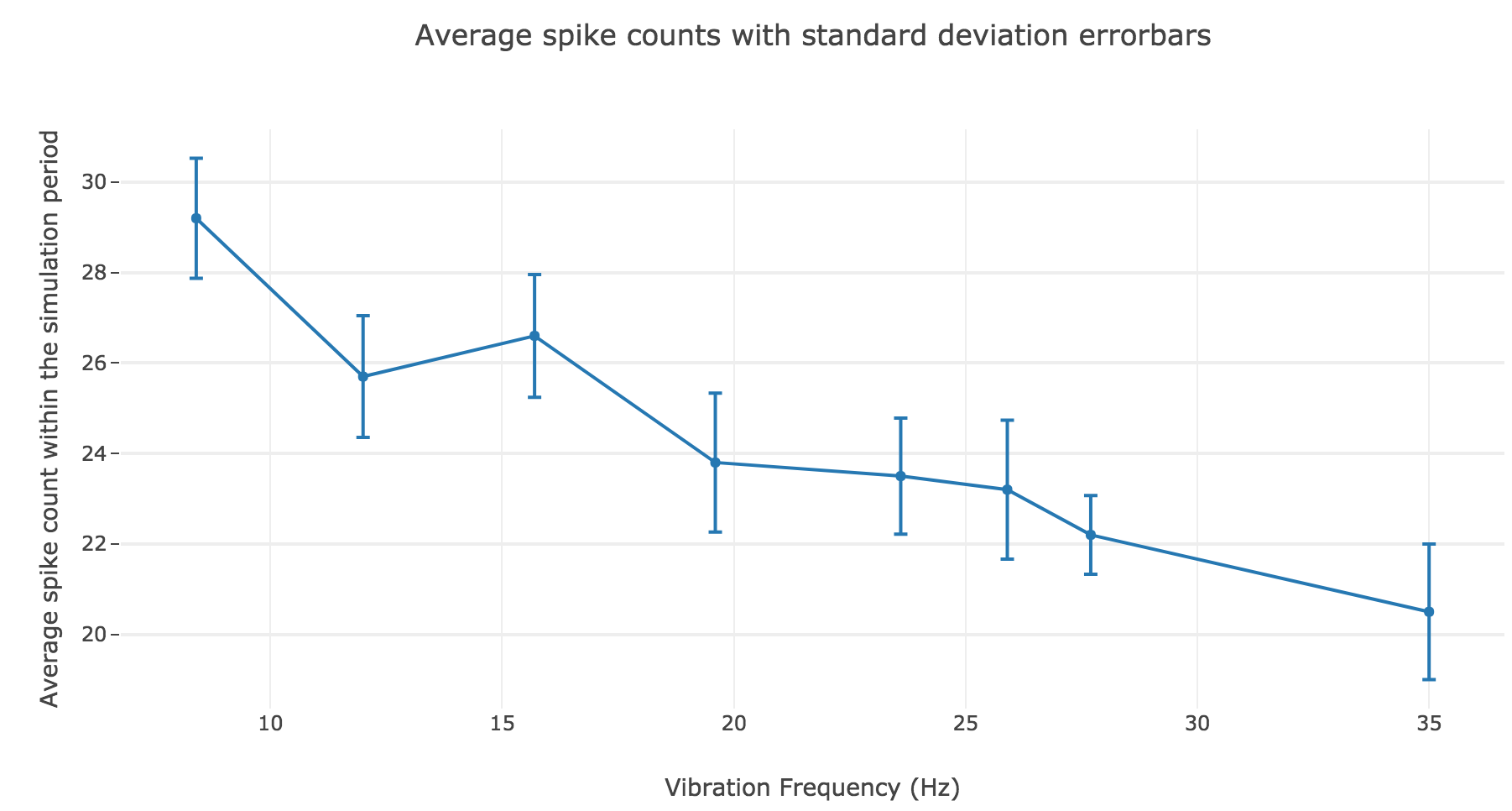Figure h.5. - Average spike count as a function of the vibration frequency with standard deviation errorbars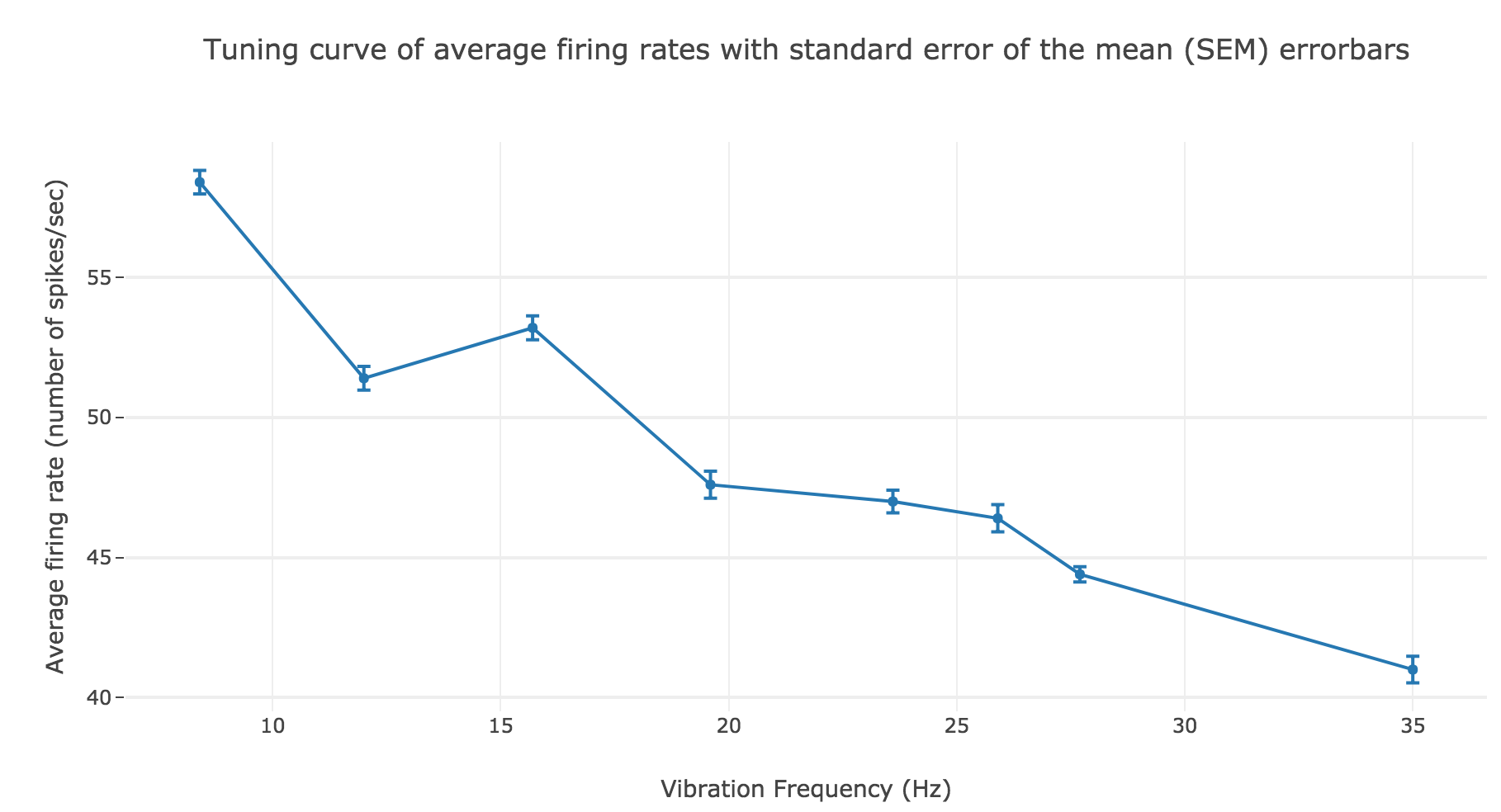Figure h.6. - Average firing rate as a function of the vibration frequency with standard error of the mean $SEM = σ/ \sqrt{N}$ errorbars

This can be explained by the fact that even though the frequency of spikes increases with the vibration frequency $f_1$, the width of the “spikes fired in a row” is decreasing (since the sinusoidal current curve is becoming thinner and thinner, the current reaches high values during a shorter and shorter time as the frequency increases). To overcome that, we would have to ensure that the width of the current waves remains the same irrespective of the frequency: this can be achieved with square waves.

If the model somehow described what happens in reality, it would mean that the current injected though the membrane of the monkey’s primary somatosensory cortex neuron when a stimulus vibrates at a frequency $f_1$ on its fingertip is:

• null outside the simulation period
• sinusoidal within the simulation period

leading to a voltage accross the membrane reaching the threshold voltage periodically (the higher the vibration frequency, the smaller the period), which in turn induces an average firing rate depending linearly on $f_1$.

Tags:

Updated: